```
[=x=-=x=-=x=-=x=-=x=-=x=-=x=-=x=-=x=-=x=-=x=-=x=-=x=-=x=-=x=-=x=-=x=]
[<<>><<>><<>><<>><<>><<>><<>><<>><<>><<>><<>><<>><<>><<>><<>><<>><<>]
[=x=-=x=-=x=-=x=-=x=-=x=-=x=-=x=-=x=-=x=-=x=-=x=-=x=-=x=-=x=-=x=-=x=]

Stardate: 20210828.0003
Location: Living Room
Input Device: Fujitsu P1610 UMPC
Audio: Chuck Mangione
Visual: stuff in living room - piano, wood floors, radio cabinet, etc.
Emotional: Unwinding from the day.

[=x=-=x=-=x=-=x=-=x=-=x=-=x=-=x=-=x=-=x=-=x=-=x=-=x=-=x=-=x=-=x=-=x=]

I put together a simple tin can mic.  Nothing fancy and it did not
take long.  I took my time so about 30 minutes.  It will be
my mic for my harmonica.  I thought about picking up one of those
bullet mics, but since I don't have cash for that right now and I do
have the parts, I figured that I would give the tin can mic a try.

The electrical parts are the same as the log of sound.  Piezo,
1/4" jack, and extension wire.  I put these inside a Murray's pomade
container, which I often reuse after using up the product for my hair.
The container is a nice size for holding the harmonica and the mic.
I hope to test it out and record something with it this weekend.

Here are some pics:

Parts and tools on my work bench.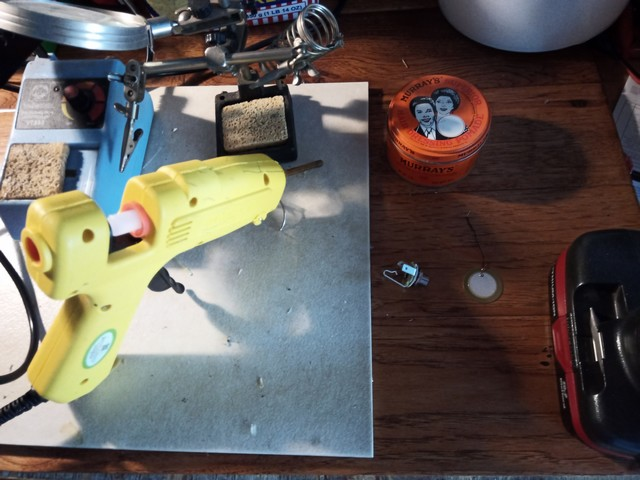Murray's can in bench vise with hole drilled for jack.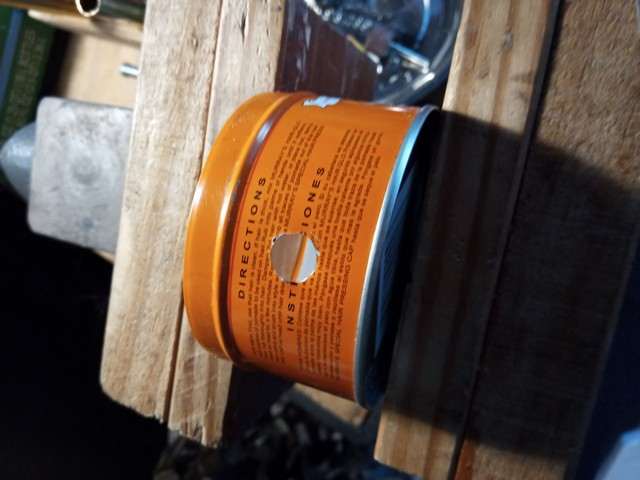Soldering piezo to scrap CAT5 wire.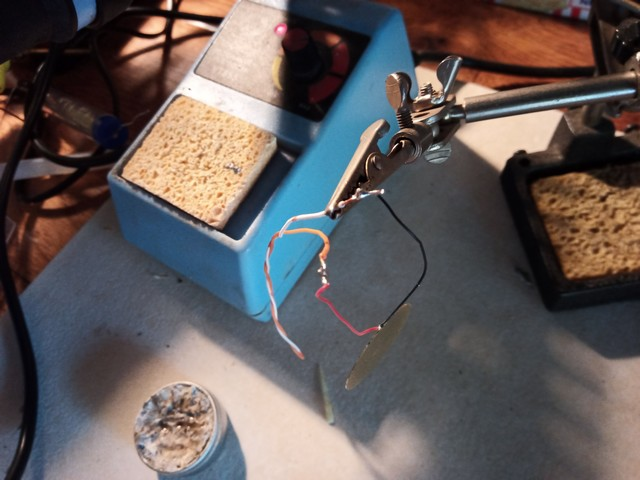Jack and piezo mounted to can.
Piezo was glued to lid with thermoplastic adhesive.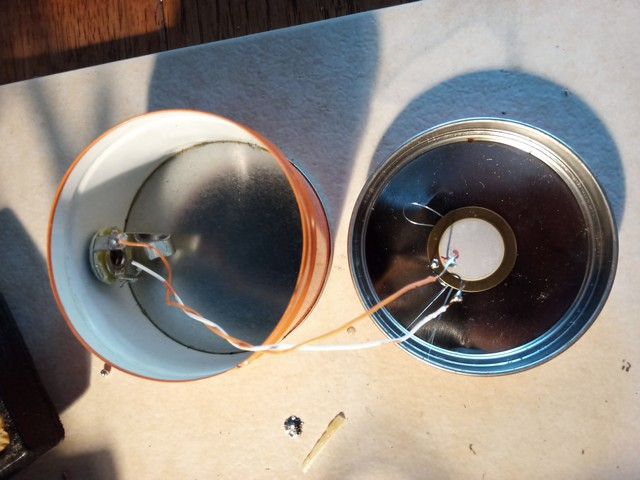Finished mic.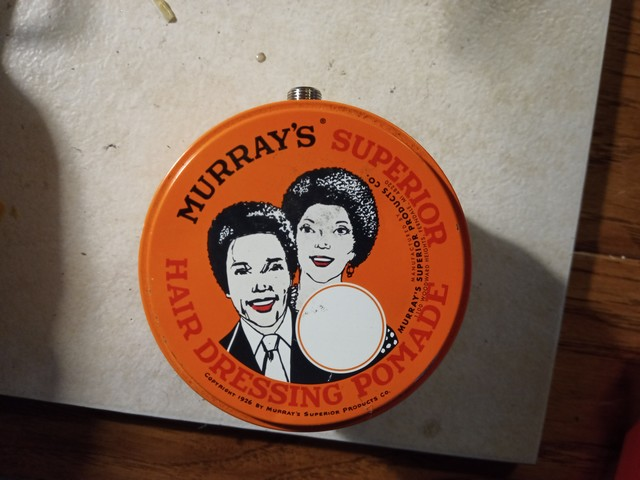Side view of mic with jack.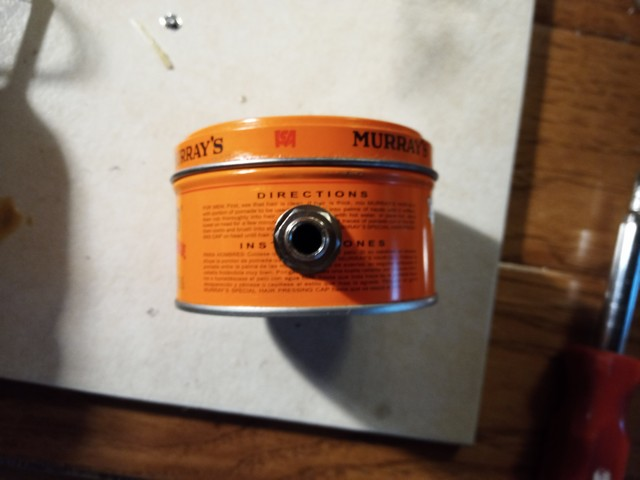Tin can mic with harmonica.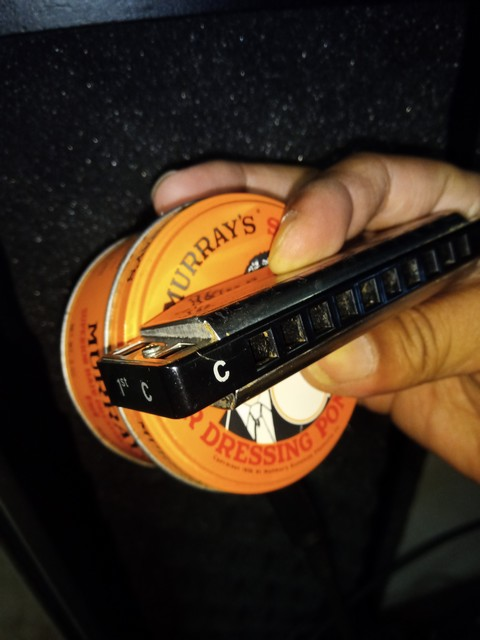[=x=-=x=-=x=-=x=-=x=-=x=-=x=-=x=-=x=-=x=-=x=-=x=-=x=-=x=-=x=-=x=-=x=]
```
.:[ HOME ]:.